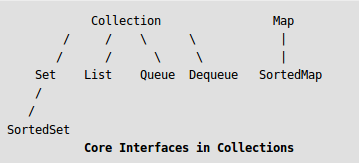# SortedMap Interface in Java with Examples

SortedMap is an interface in collection framework. This interface extends Map interface and provides a total ordering of its elements (elements can be traversed in sorted order of keys). Exampled class that implements this interface is TreeMap.The main characteristic of a SortedMap is that, it orders the keys by their natural ordering, or by a specified comparator. So consider using a TreeMap when you want a map that satisfies the following criteria:

• null key or null value are not permitted.
• The keys are sorted either by natural ordering or by a specified comparator.

Methods of SortedMap:

1. subMap(K fromKey, K toKey): Returns a view of the portion of this Map whose keys range from fromKey, inclusive, to toKey, exclusive.
2. headMap(K toKey): Returns a view of the portion of this Map whose keys are strictly less than toKey.
3. tailMap(K fromKey): Returns a view of the portion of this Map whose keys are greater than or equal to fromKey.
4. firstKey(): Returns the first (lowest) key currently in this Map.
5. lastKey(): Returns the last (highest) key currently in this Map.
6. comparator(): Returns the Comparator used to order the keys in this Map, or null if this Map uses the natural ordering of its keys.
7. values(): Returns a Collection view of the values contained in this map.
8. keySet(): Returns a Set view of the keys contained in this map.
9. entrySet(): Returns a Set view of the mappings contained in this map.

Code for SortedMap:

```public interface SortedMap extends Map
{
Comparator comparator();
SortedMap subMap(K fromKey, K toKey);
SortedMap tailMap(K fromKey);
K firstKey();
K lastKey();
}
```

 `// Java code to demonstrate SortedMap ` `import` `java.util.Iterator; ` `import` `java.util.Map; ` `import` `java.util.Set; ` `import` `java.util.SortedMap; ` `import` `java.util.TreeMap; ` ` `  `public` `class` `SortedMapExample ` `{ ` `    ``public` `static` `void` `main(String[] args) ` `    ``{ ` `        ``SortedMap sm = ` `                    ``new` `TreeMap(); ` `        ``sm.put(``new` `Integer(``2``), ``"practice"``); ` `        ``sm.put(``new` `Integer(``3``), ``"quiz"``); ` `        ``sm.put(``new` `Integer(``5``), ``"code"``); ` `        ``sm.put(``new` `Integer(``4``), ``"contribute"``); ` `        ``sm.put(``new` `Integer(``1``), ``"geeksforgeeks"``); ` `        ``Set s = sm.entrySet(); ` ` `  `        ``// Using iterator in SortedMap ` `        ``Iterator i = s.iterator(); ` ` `  `        ``// Traversing map. Note that the traversal ` `        ``// produced sorted (by keys) output . ` `        ``while` `(i.hasNext()) ` `        ``{ ` `            ``Map.Entry m = (Map.Entry)i.next(); ` ` `  `            ``int` `key = (Integer)m.getKey(); ` `            ``String value = (String)m.getValue(); ` ` `  `            ``System.out.println(``"Key : "` `+ key + ` `                            ``"  value : "` `+ value); ` `        ``} ` `    ``} ` `} `

Output:

```Key : 1  value : geeksforgeeks
Key : 2  value : practice
Key : 3  value : quiz
Key : 4  value : contribute
Key : 5  value : code
```

This article is contributed by Pratik Agarwal. If you like GeeksforGeeks and would like to contribute, you can also write an article using contribute.geeksforgeeks.org or mail your article to contribute@geeksforgeeks.org. See your article appearing on the GeeksforGeeks main page and help other Geeks.My Personal Notes arrow_drop_up

Improved By : 1rajarshimondal

Article Tags :
Practice Tags :

6

Please write to us at contribute@geeksforgeeks.org to report any issue with the above content.